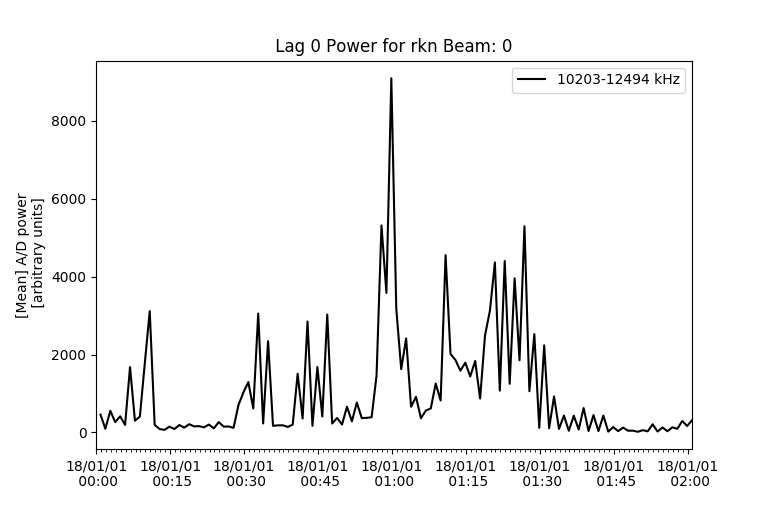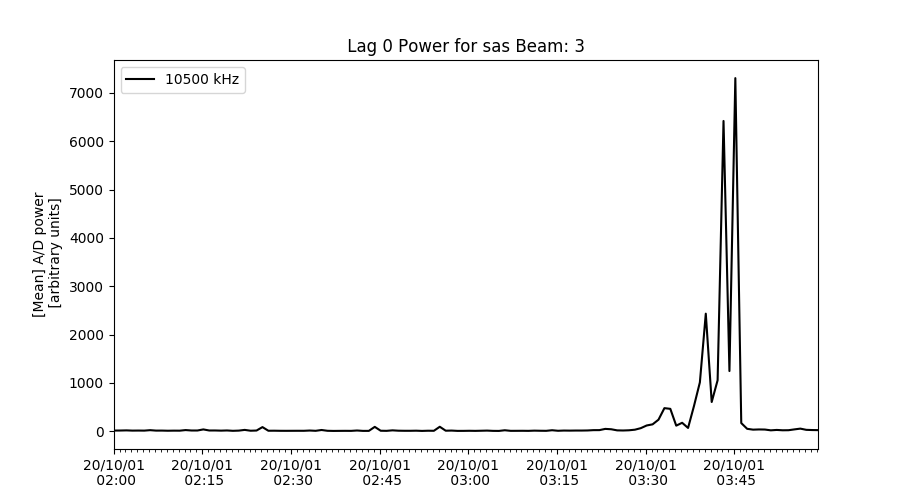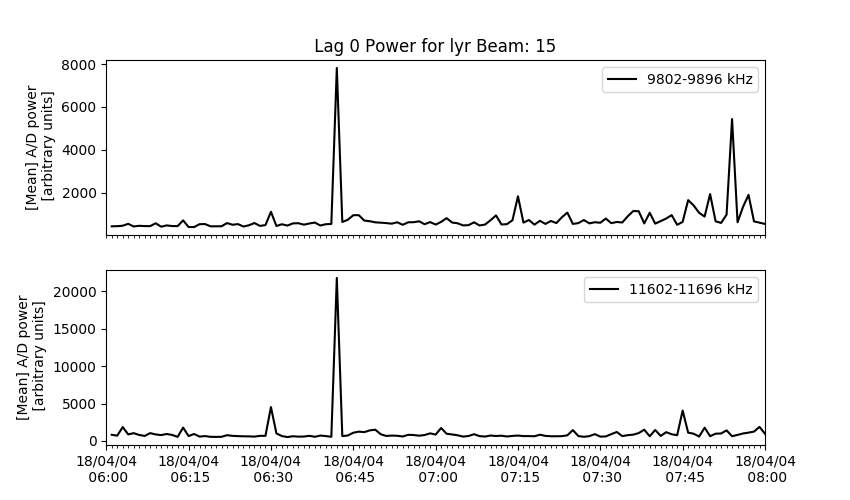### Statistical Power Plots

This function will calculate and plot a statistic of the lag-0 power of each record as a function of time.

This function applies the statistical function (ex. numpy.mean) to the pwr0 vector (lag-0 power for each range) for each record before plotting the results from all records chronologically.

This code can be used to study background interference in rawacf data when the radar has been operating in a receive-only mode such as "politescan" (cpid -3380), or during periods without any obvious coherent scatter returns from any range.

Basic code to plot lag 0, will plot the mean of `pwr0` for all frequencies at beam 0, from a RAWACF file would look like:

``````import matplotlib.pyplot as plt
import pydarn

file = "20180101.0000.01.rkn.rawacf"

pydarn.Power.plot_pwr0_statistic(rawacf_data)
plt.show()
``````Parameter Action
beam_num=0 beam number to plot
min_frequency minimum Frequency (inclusive) to plot
max_frequency maximum Frequency (inclusive) to plot
split_frequency specific frequency to look for or split between
statistical_method=np.mean the statistical calculation to apply to the `pwr0` array

If you want display only one frequency set `min_frequency = max_frequency`:

``````import pydarn
import matplotlib.pyplot as plt

rawacf_file = '20201001.0200.00.sas.0.rawacf.hdf5'
pydarn.Power.plot_pwr0_statistic(rawacf_data, beam_num=3, min_frequency=10500, max_frequency=10500)
plt.show()
``````Warning

If the radar is running non-normal scan mode then the this may produce a blank plot or raise an error of No Data Found. Also, ROS superDARN radars will vary slightly in a frequency thus causing issues for picking a specific frequency.

To compare data above and below a specific frequency, you can compare by using the `split_frequency` option:

``````import pydarn
import matplotlib.pyplot as plt

rawacf_file = 'data/20180404.0601.00.lyr.rawacf'Depending on the mode that is being run, type of radar system, and the frequency range you may get this error `plot_exceptions.NoDataFoundError`. Make sure you know the frequency range for your data as there might be data at a different frequency range, the parameters just need to be tweaked a bit.